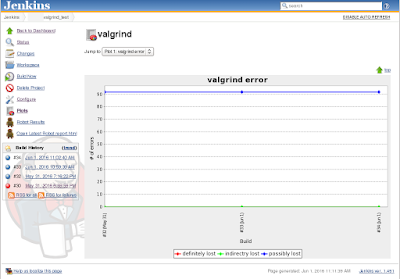## 2016-06-01

I use Jenkins  for a nightly automated test. In the test I use valgrind  for memory leak detection and has some graph of number of errors. So I would like to use plot plugin .

From the example plot image, it is clear we can have a graph with multiple lines. However, how to do it was a bit ambiguous to me from the web page, the example perl script, and the help (in the plot plugin).

The key idea is I need to have multiple data files for multiple lines.

For example, in the build I made the following property data files. Each file represents one data line.

• valgrind_trunk_result.definitely.property
• valgrind_trunk_result.indirectly.property
• valgrind_trunk_result.possibly.property

The contents of each file is only one line. For example, valgrind_trunk_result.definitely.property is:

YVALUE=0

This file is put under the directory of \${WORKSPACE} where the environment variable "WORKSPACE" is given by jenkins.

Figure 1 shows my plot plugin setup in the jenkins configure. There are three data series and each of them has each file.Figure 1: Plot plugin configuration in Jenkins

You see there are multiple lines in Figure 2. (Actually there are three lines in the graph, but the first and second data are the same, so these lines overlapped, thus you see only two lines.)Fugure 2: Plot data with multiple data lines